# 7+ Simple Metric Conversion Chart Templates

The Simple Metric Conversion Chart Templates is the basic and simplest of all and consists of only length (meter), mass (gram), volume (liter) and time (second) as it’s metric units. This chart can be widely used by numerous people in a majority of professions owing to its nature of simple conversions. This conversion chart ensures that you need not invest a lot of time in either frisking through a large amount of unnecessary information or making complicated calculations.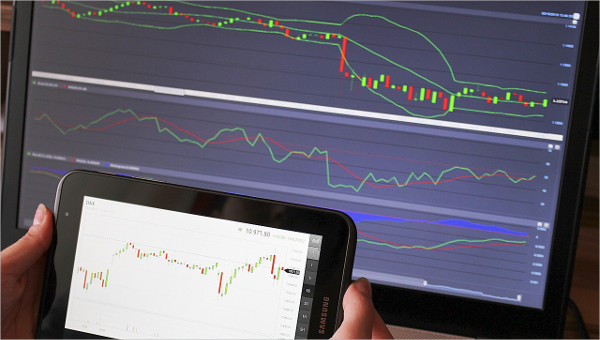## Science Made Simple Metric Conversion Chart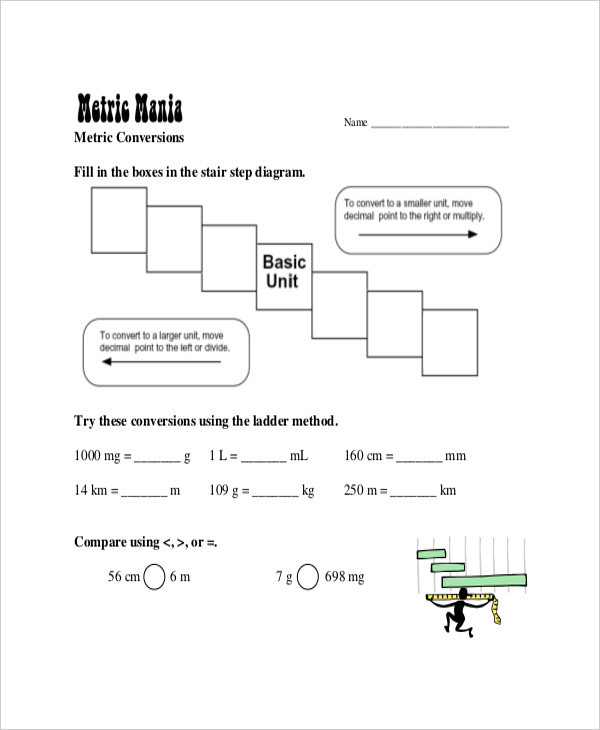sciencespot.net

These conversion Chart Templates is exclusively used by both basic and advanced students of science and consists of metric units such as area, currency, distance, length, power, pressure, speed, temperature, time, volume and weight.

## Simple Conversion Chart For Metric System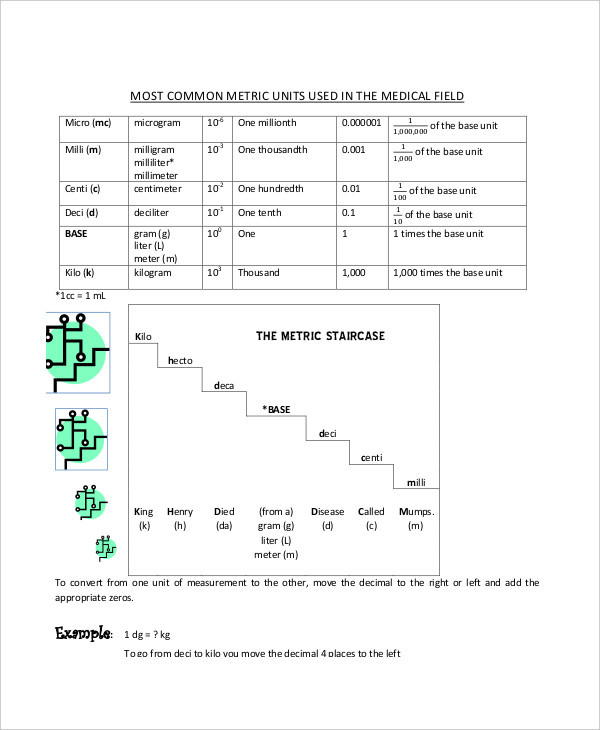laniertech.edu

The Simple conversion chart is the simplest metric conversion reference chart and is predominantly used by the students of primary level and by individuals who require referring to basic conversions for their work.

## Simple Metric Weight Conversion Chart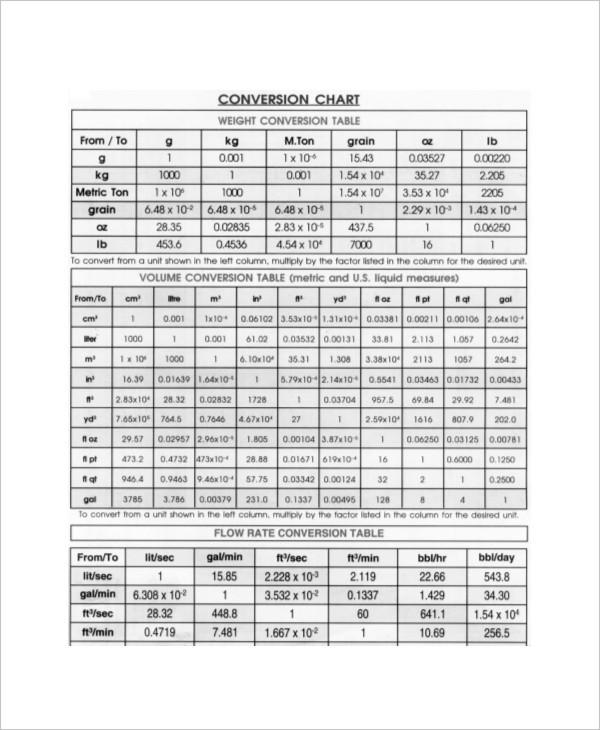blulux.com

This conversion chart is exclusively referred for weight conversions and deals in the imperial units such as kilograms, stones, ounces and pounds to measure mass and weight of living beings and objects.

## Simple Imperial Metric Conversion Chart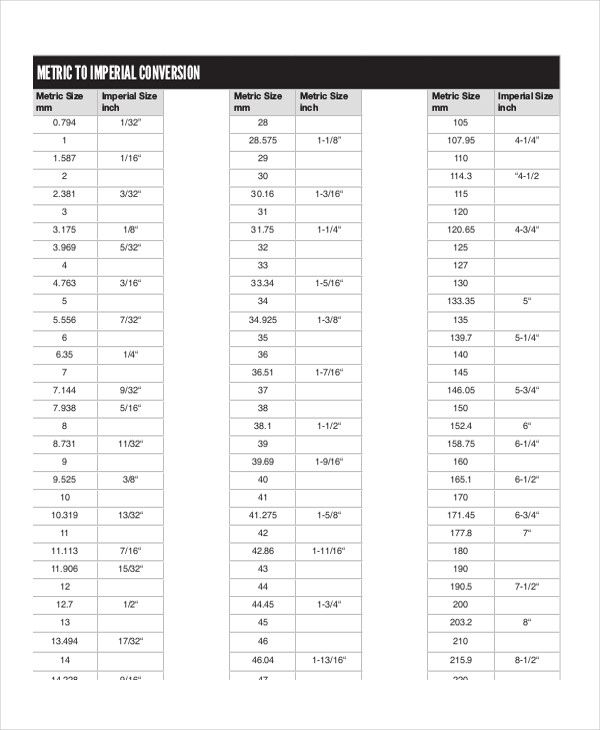interlloy.com.au

The Imperial Metric Conversion Chart is a simple conversion chart much like the US Conversion chart which is used to convert and measure units such as length, area, volume, mass and temperature.

## Simple Metric Conversion Chart Length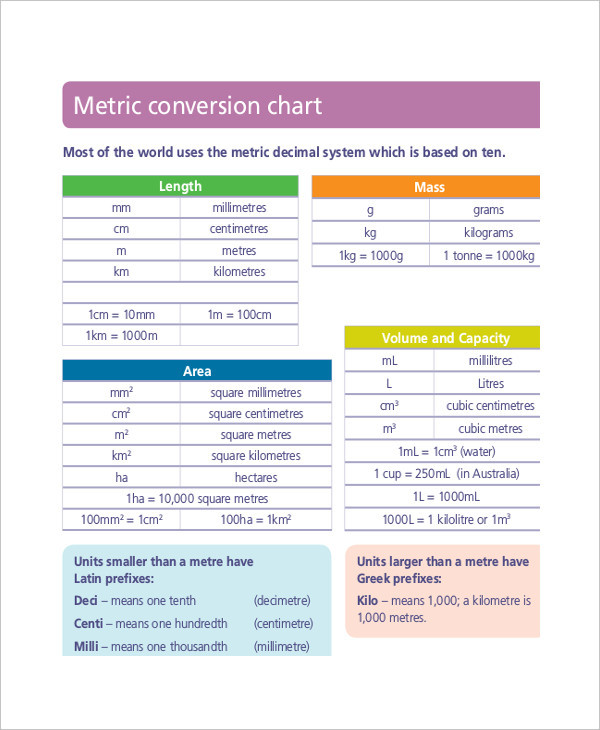det.nsw.edu.au

The Length Conversion Chart is used by one and all to convert and calculate the units of length and distance such as centimeters, inches, feet, yard, meters, miles and kilometers.

## Simple Height & Weight Metric Conversion Chart Template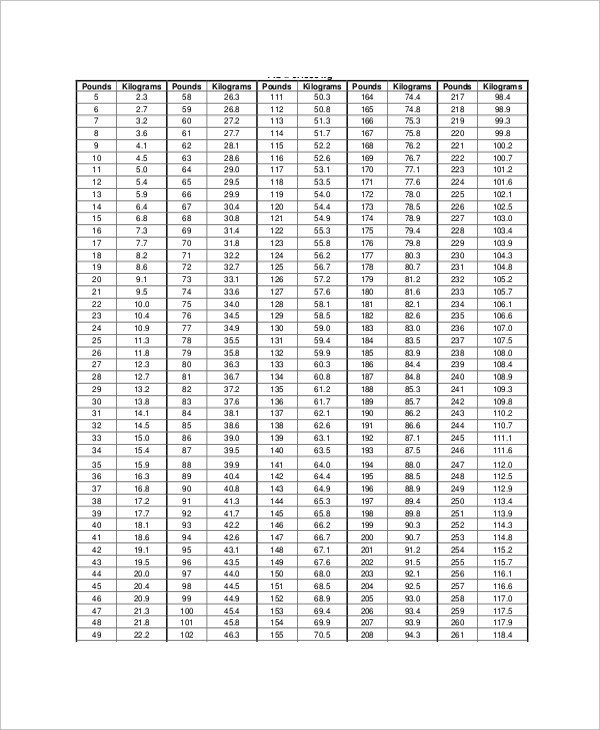calgarylabservices.com

## Simple Metric Unit Conversion Chart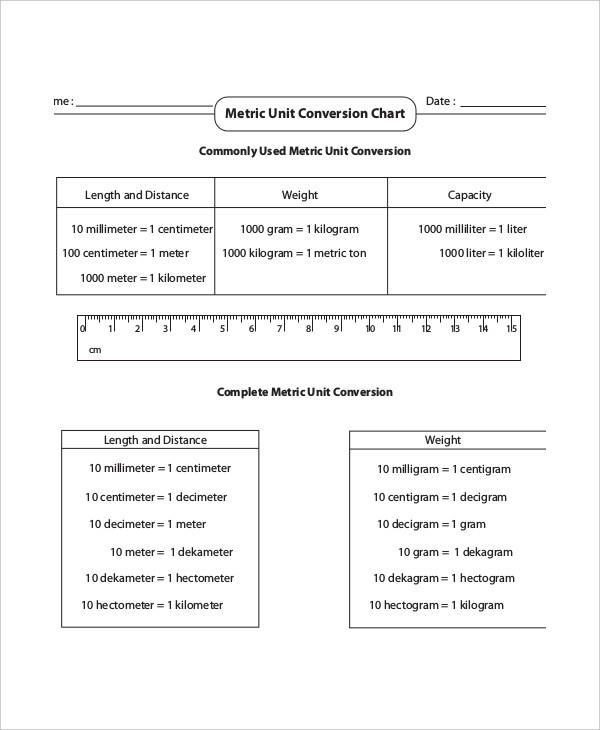mathworksheets4kids.com

### > How to use the Metric System Conversion Chart?

The Simple Metric system conversion chart comprises of the simplest of units of measurements such as area, currency, distance, length, power, pressure, speed, temperature, time, volume and weight. The chart is pretty simple to use and refer and you can accommodate this into your daily work references as it does not demand the use of complex calculations to arrive at the desired value. These charts are so easy and useful that you can also print them out and can hang them at your work desk for easy reference. You can also see Height Weight Chart Templates.

### > What are the advantages of the Metric System Conversion Chart?

There are myriad advantages of the Metric System conversion charts especially the ones which are basic and easy to use. These simple to refer documents are extremely handy for individuals who do not practice Mathematics professionally or academically but require converting units of measurement every now and then for work purposes as well as personal reasons.

Additionally, the availability of defined metric system conversion charts such as length and weight ensures that you get a customized metric system conversion chart which is devoid of any additional information in the form of other units of measurements and only caters to your individual needs. You can also see Metric System Conversion Chart Templates.

The Simple Metric Conversion chart is a simple unit conversion document containing the list of the basic units of system conversion such as area, distance, time, speed, temperature, pressure, electric current and light which you can download, save for later and use to calculate values within a given minimal time.